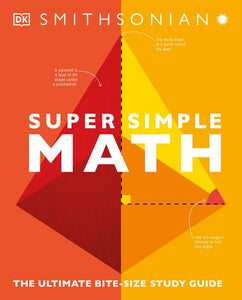# Super Simple Math

• \$28.99

All the core curriculum math topics in one super simple book - an accessible and indispensable guide for students, parents, and educators

Covering topics from probability to statistics, algebra to geometry, this study guide is what every young, budding mathematician needs to succeed in math - both at home and in school.

This math study book is the perfect support for coursework, homework, and exam revision for 11-16 year olds. Inside students will find:

- Key concepts shown in visual form and summarized in a single page
- Important aspects of each topic explained with step-by-step instructions and diagrams
- Key fact boxes that break down core concepts and make the material easier to memorize
- "How it works" and "Look closer" boxes explain essential ideas and work through problems with simple graphics
- Practice questions to help students test their understanding of important topics
x